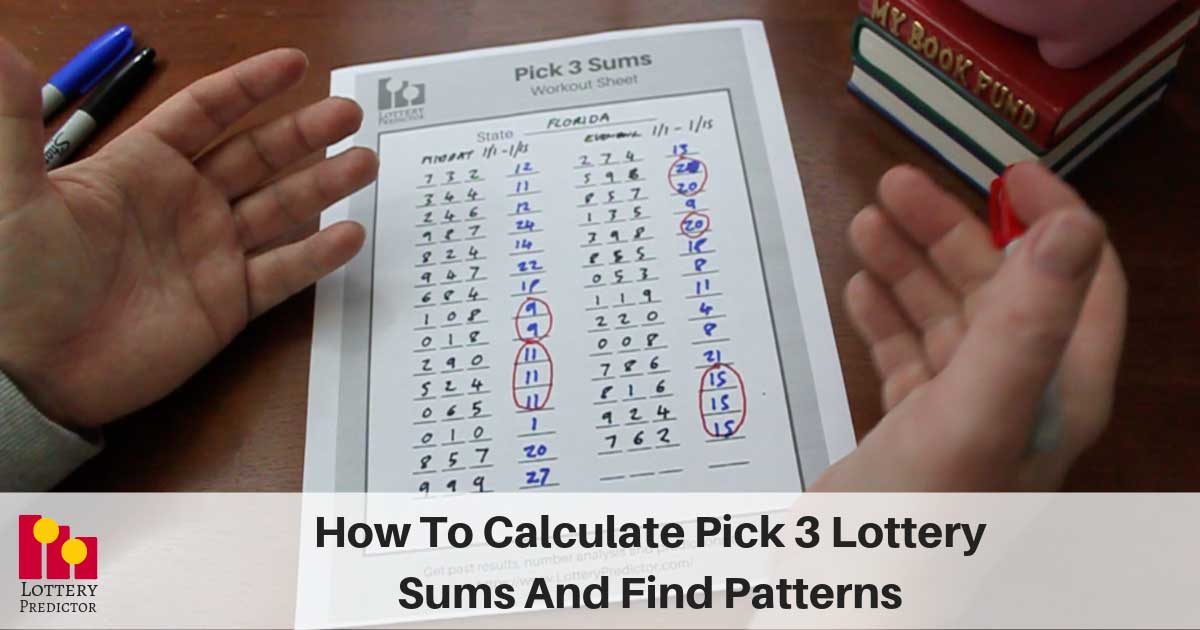#### How To Calculate Pick 3 Lottery Sums And Find Patterns##### How To Calculate Pick 3 Lottery Sums And Find Patterns

2019-01-16

Lottery number sums can be one of the key foundations to finding patterns in lottery drawings and can help with picking your next set of numbers to play. While they may seem complex in the face of it, they are actually very easy to calculate and use. The video below will show you how to calculate sums for the Pick 3 lottery and then some of the techniques you can use to find patterns in them.

If you wish to skip the video, here are the basics. All you have to do to calculate the sum of a pick 3 number is break it down into its individual numbers. For example

327 would be 3, 2 and 7

You then take these individual numbers and add them together to get the sum.

3 + 2 + 7 = 12

So the sum of the draw 327 would be 12.

To find patterns with these numbers, calculate the sum for several drawings in a row. Then you can start tp search for patterns such as sequential numbers, odds and even patterns, number groupings etc. Find the pattern that is currently working for your state and then follow that pattern.

If you enjoyed the video make sure you like and subscribe to the channel and also check out the other videos.